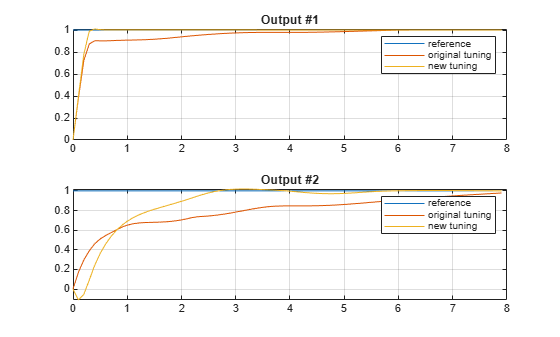Documentation

## Adjust Input and Output Weights Based on Sensitivity Analysis

This example shows how to compute numerical derivatives of a closed-loop cumulated performance index with respect to weights and use them to improve model predictive controller performance.

### Define Plant Model

Create a state-space model for the plant.

`plant = ss(tf({1,1,2;1 -1 -1},{[1 0 0],[1 0 0],[1 1];[1 2 8],[1 3],[1 1 3]}),'min');`

### Design MPC Controller

Create an MPC controller with a specified sample time `Ts`, prediction horizon `p`, and control horizon `m`.

```Ts = 0.1; p = 20; m = 3; mpcobj = mpc(plant,Ts,p,m);```
```-->The "Weights.ManipulatedVariables" property of "mpc" object is empty. Assuming default 0.00000. -->The "Weights.ManipulatedVariablesRate" property of "mpc" object is empty. Assuming default 0.10000. -->The "Weights.OutputVariables" property of "mpc" object is empty. Assuming default 1.00000. ```

Set constraints on manipulated variables and their rates of change.

```for i = 1:3 mpcobj.MV(i).Min = -2; mpcobj.MV(i).Max = 2; mpcobj.MV(i).RateMin = -4; mpcobj.MV(i).RateMax = 4; end```

Set weights on output variables.

`mpcobj.Weights.OutputVariables = [1 1];`

Set weights on the rates of change of the manipulated variables.

`mpcobj.Weights.ManipulatedVariablesRate = [.1 .1 .1];`

Keep the weights on manipulated variables remain at their default values, `[0 0 0]`.

### Performance Evaluation Setup

The default closed-loop performance is expressed through a set of weights that reflect the desired closed-loop behavior. The weights are contained in a structure with the same fields as the `Weights` property of an MPC object.

`PerformanceWeights = mpcobj.weights;`

In this example, we make output weights more important than weights on MV rates in evaluating closed-loop performance.

```PerformanceWeights.OutputVariables = [100 100]; PerformanceWeights.ManipulatedVariablesRate = [1 1 1];```

Note that `PerformanceWeights` is only used in the cumulated performance index computation. It is not related to the weights specified inside the MPC controller object.

### Setup Simulation Options

In this example, we only inspect the setpoint tracking scenario for the sensitivity analysis.

```Tstop = 80; % time steps to simulate r = ones(Tstop,1)*[1 1]; % set point signals v = []; % no measured disturbance simopt = mpcsimopt; simopt.PlantInitialState = zeros(8,1);```

### Compute Sensitivities

`[J1, Sens1] = sensitivity(mpcobj, 'ISE', PerformanceWeights, Tstop, r, v, simopt);`
```-->Converting model to discrete time. Assuming no disturbance added to measured output channel #1. -->Assuming output disturbance added to measured output channel #2 is integrated white noise. -->The "Model.Noise" property of the "mpc" object is empty. Assuming white noise on each measured output channel. ```
```disp('') disp('--------------')```
```-------------- ```
`disp('Sensitivity analysis')`
```Sensitivity analysis ```
`disp('--------------')`
```-------------- ```
```disp('') fprintf('Output weights: dJ/dWy = [%g, %g]\n',Sens1.OutputVariables);```
```Output weights: dJ/dWy = [-27345.7, 27166] ```
`fprintf('Input weights: dJ/dWu = [%g, %g, %g]\n',Sens1.ManipulatedVariables);`
```Input weights: dJ/dWu = [3.33756, -125.827, -35.1066] ```
`fprintf('Input-rate weights: dJ/dWdu = [%g, %g, %g]\n',Sens1.ManipulatedVariablesRate);`
```Input-rate weights: dJ/dWdu = [-7.30066, 10250.2, -8369.89] ```
`disp('--------------')`
```-------------- ```
`disp('')`

Since we always want to reduce closed-loop cumulated performance index `J`, in this example the derivatives with respect to output weights show that the weight on `y1` should be increased, as the corresponding derivative is negative, while the weight on `y2` should be decreased.

`mpcobj_new = mpcobj;`

Sensitivity less than 0 suggests increasing output weight from 1 to 2.

`mpcobj_new.Weights.OutputVariables(1) = 2;`

Sensitivity greater than 0 suggests decreasing output weight from 1 to 0.2.

`mpcobj_new.Weights.OutputVariables(2) = 0.2;`

Note that the sensitivity analysis only tells you which direction to change the parameters, not how much. Trial and error is expected.

### Verify Performance Changes

```[y1, t1] = sim(mpcobj, Tstop, r, v, simopt); [y2, t2] = sim(mpcobj_new, Tstop, r, v, simopt);```
```-->Converting model to discrete time. Assuming no disturbance added to measured output channel #1. -->Assuming output disturbance added to measured output channel #2 is integrated white noise. -->The "Model.Noise" property of the "mpc" object is empty. Assuming white noise on each measured output channel. ```
```h = figure; subplot(211) plot(t2,r(:,1),t1,y1(:,1),t2,y2(:,1));grid legend('reference','original tuning','new tuning') title('Output #1') subplot(212) plot(t2,r(:,2),t1,y1(:,2),t2,y2(:,2));grid legend('reference','original tuning','new tuning') title('Output #2')```### Verify Cumulated Performance Index is Reduced

Recompute just the cumulated performance index using the same performance measure.

`J2 = sensitivity(mpcobj_new, 'ISE', PerformanceWeights, Tstop, r, v, simopt);`
```-->Converting model to discrete time. Assuming no disturbance added to measured output channel #1. -->Assuming output disturbance added to measured output channel #2 is integrated white noise. -->The "Model.Noise" property of the "mpc" object is empty. Assuming white noise on each measured output channel. ```
`fprintf('Previous Cumulated Performance Index J1 = %g\n',J1);`
```Previous Cumulated Performance Index J1 = 128645 ```
`fprintf('New Cumulated Performance Index J2 = %g\n',J2);`
```New Cumulated Performance Index J2 = 116234 ```

Note that the absolute value of the cumulated performance index is not important.

### Use a User-Defined Performance Function

This is an example of how to write a user-defined performance function used by the `sensitivity` method. In this example, custom function `mpc_performance_function.m` illustrates how ISE performance index is implemented.

```J3 = sensitivity(mpcobj,'mpc_performance_function',Tstop,r,PerformanceWeights); fprintf('User Defined Cumulated Performance Index J3 = %g (same as J1).\n',J3);```
```User Defined Cumulated Performance Index J3 = 128645 (same as J1). ```